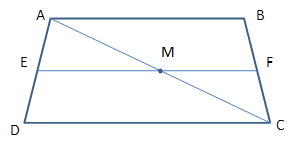Chapter 4.4, Problem 37EElementary Geometry For College St...

7th Edition
Alexander + 2 others
ISBN: 9781337614085

Solutions

Chapter
SectionElementary Geometry For College St...

7th Edition
Alexander + 2 others
ISBN: 9781337614085
Textbook Problem

For exercises 36 and 37, E F ¯ is the median of trapezoid ABCD in the figure above.Suppose that E M = 7.1             a n d             M F = 3.5 Find: (a) AB (b) EF (c) DC (d) Whether E F = 1 2 ( A B + D C )Exercises 35-37

To determine

(a)

To Find:

AB

Explanation

Consider the following diagram.

Given: EM = 7.1 and MF = 3.5.

Consider the triangle ΔABC.

We know that the line segment that joins the midpoints of two sides of a triangle is parallel to the third side and has a length equal to one half the length of the third side.

Here, the line segment MF joins the midpoints of AC¯

To determine

(b)

To Find:

DC

To determine

(c)

To Find:

EF

To determine

(d)

To Find:

Whether EF=12(AB+DC)

Still sussing out bartleby?

Check out a sample textbook solution.

See a sample solution

The Solution to Your Study Problems

Bartleby provides explanations to thousands of textbook problems written by our experts, many with advanced degrees!

Get Started

Find the limit. limxln(sinx)

Single Variable Calculus: Early Transcendentals, Volume I

Solve the equations in Exercises 112 for x (mentally, if possible). x3=1

Finite Mathematics and Applied Calculus (MindTap Course List)

Consider the data 10, 12, 12, 8, 30, 12, 8, 10, 5, 23. 2. Find the median.

Mathematical Applications for the Management, Life, and Social Sciences

True or False: is a convergent series.

Study Guide for Stewart's Single Variable Calculus: Early Transcendentals, 8th